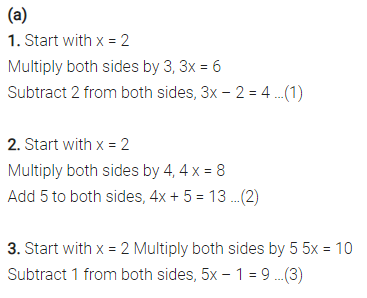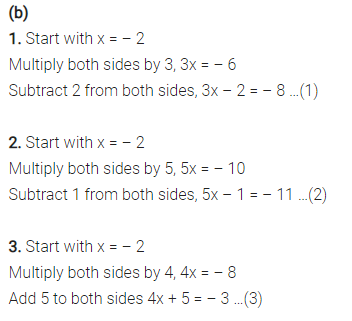# (a) Construct 3 equations starting with x = 2 (b) Construct 3 equations starting with x = – 2

(a) Construct 3 equations starting with x = 2
(b) Construct 3 equations starting with x = – 2.